Development of low power Dynamic threshold PCS System | OMICS International
Journal of Electrical & Electronic Systems

# Development of low power Dynamic threshold PCS System

Pawan Whig1* and Syed Naseem Ahmad2

1Department of Electronics and Communication, Bhagwan Parshuram College of Engineering and Technology Rohini, Delhi, India

2Head of Department, Department of Electronics and Communication Engineering, Jamia Millia Islamia, New Delhi 110025, India

*Corresponding Author:
Pawan Whig
Assistant Professor
Department of Electronics and Communication Engineering
Bhagwan Parashuram Institute of Technology
New Delhi-110025, India
E-mail: [email protected]

Received Date: August 28, 2014; Accepted Date: September 15, 2014; Published Date: September 26, 2014

Citation: Whig P, Ahmad SN (2014) Development of Low Power Dynamic Threshold PCS System. J Electr Electron Syst 3:131. doi:10.4172/2332-0796.1000131

Copyright: © 2014 Whig P, et al. This is an open-access article distributed under the terms of the Creative Commons Attribution License, which permits unrestricted use, distribution, and reproduction in any medium, provided the original author and source are credited.

Visit for more related articles at Journal of Electrical & Electronic Systems

#### Abstract

Real-time reception of sensor signals is one of the most significant operations in analog signal processing. Driven by low-power and low-voltage requirements for integrated mixed signal portable applications, this paper propose a novel low power Dynamic Threshold Photo Catalytic Sensor (DTMOS) system to work efficiently below lower bound of power supply independent of substrate bias effect. Another important feature of DTMOS is that the fabrication of circuits based on DTPCS is less complex and more economical. The SPICE simulations were performed with 120 nm technology parameters and results verify the performance of the circuit. The proposed system has high linearity and simple structure hence it is suitable for high-performance and low-power analog VLSI applications. The main reason of employing a readout circuit to PCS circuitry, is the fact that the fluctuation of O2 influences the threshold voltage, which is internal parameter of the FET and can manifest itself as a voltage signal at output but as a function of the transconductance gain. The trans- conductance is a passive parameter and in order to derive voltage or current signal from its fluctuations the sensor has to be attached to readout circuit. This circuit provides high sensitivity to the changes in percentage of O2 in the solution.

#### Keywords

Sensor; Dynamic Threshold Photo catalytic Sensor (DTMOS); Low power; VLSI

#### Introduction

Water is indispensable source of our life. According to Central Pollution Control Board, 90% of the water supplied in India to the town and cities is polluted, out of which only 1.6% gets treated. Therefore, water quality management is fundamental for the human welfare. The statistical regression analysis has been found to be a highly useful tool for correlating different parameters. Correlation analysis measures the closeness of the relationship between chosen independent and dependent variables. If the correlation coefficient is nearer to +1 or -1, it shows the probability of linear relationship between the variables x and y . This way analysis attempts to establish the nature of the relationship between the variables and thereby provides a mechanism for prediction or forecasting.

In the field of artificial waste water analysis and COD determination, TiO2 sensors have large number of potential applications due to their highly efficient photo activity, steadiness and least cost. From the earlier times TiO2 is used as white pigment which is safe for human use. The results achieved by the use of TiO2 Photo Catalytic Sensor are in good agreement with those from the conventional Dichromate method. Very complex and bulky set up is needed for the conventional method used for Photo Catalytic Sensor applications and consumes a plenty of time for computation. To provide a solution to these problems and make the application faster, Whig and Ahmad developed a Simulation Program with Integrated Circuit Emphasis (SPICE) model for Photo Catalytic Sensor . With the advancement in semiconductor technology development of sensors has become easy due to advantages of low power, high speed signal processing capabilities. CAD tools are an added advantage as they provide a method for simulation and synthesis of semiconductor sensors. By using the SPICE model, the size and power of the overall system can be minimized thus increasing the reliability of the system.

In the Modern electronics there is a great demand for low power and high performance digital system . The Low power concept in semiconductor industries relies on a basic concept of power supply scaling. Since reduction in power supply below 3Vt will degrade the speed of circuit. Hence scaling of power supply should be done along with threshold voltage reduction. The threshold voltage cannot be reduced by lower bound a certain lower limit. The lower limit of threshold voltage can be set by keeping the value of offset leakage current that can be withstand by the circuit. To increase the flexibility of operation of device to work efficiently below lower bound of power supply a new technique DTPCS with highest Vt at zero bias and lower limit at Vgs = Vdd is proposed in the rest of the paper.

#### Photocatalysis Process

The process of photocatalysis is a proficient method for degrading organic compounds. Various literatures are available on the different mechanisms and equations involved in the process for gaining a better knowledge . The semiconductor material consists of two bands which are valence band and conduction band. The energy gap between these two bands is known as band gap given by Eg. The electrons from the valence band jump to conduction band which may be empty when a light of energy higher than band gap energy falls on the semiconductor material. Holes are left behind in the valence band due to excitation of electrons to higher energy band. These holes on reaching the surface of the organic molecule reacts with water to give OH- radicals for oxidizing the organic pollutants. The dissolved oxygen in the molecular form acts as a scavenger of the photogenerated electrons and forms a superoxide radical ion. Titanium oxide has the ability to cause photooxidative destruction of the organic pollutants and is non-corrosive in nature due to which it is used as a catalyst in the process . The oxygen content in any given sample can be determined by observing the change in dissolved oxygen concentration during the process of photocatalysis.

The Photo Catalytic Sensor (PCS) senses the changes in the oxygen concentration and its voltage levels change as an indication. The cross section of the PCS is shown in Figure 1.

PCS (Photo catalytic sensor)

The SPICE model for PCS is given in . It is basically a MOSFET having structural difference in which the gate terminal is kept inside the solution and diffusion and quantum capacitances are added to explain the effect of Helmholtz and diffusion layer . The cross section of PCS is shown in Figure 2.

The threshold voltage equation for the PCS model is given in equation 1: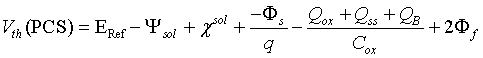(1)

Where Ψsol is an input parameter of the equation which is dependent on the concentration of O2 in the solution and surface dipole potential (χsol). Here ERef is the constant reference electrode potential. For different concentrations of O2, different V-I curves for PCS can be plotted. Ψsol is a function of O2 and as the saturation cut-off current Ids increases the value of the oxygen concentration level decreases. The circuit for PCS as given in is shown in Figure 3.

Here CM is the resultant of Cox and Cq which are oxide and quantum capacitances respectively. The equivalent capacitance CM is given in equation 2.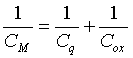(2)

The drain current equation in non-saturation mode for PCS is given in equation 3.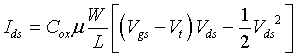(3)

where

Cox = Oxide capacitance per unit area,

μ = Electron mobility of the channel,

W = Channel width

L = Length of the Channel

The curves between Ids and Vds is shown in Figure 4 for different values of oxygen contet.

#### Device Description and Analysis

The PCS generates potential proportional to activity of detected oxygen ion. Potential in PCS is measured against the reference electrode. The Potentiometric method previously used had a serious limitation that for multiple sensors network multiple reference electrodes are needed  also the system is suffer from body bias effect. To measure the change in the concentration of dissolved oxygen through a corresponding shift in the device threshold voltage independent of substrate bias effect using DTMOS PCS is shown in Figure 5.

The threshold voltage of DTMOS transistor is given as: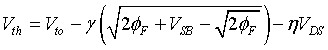(4)

Where to V threshold voltage is when substrate bias voltage is zero γ is a body bias coefficient

φF is surface potential.

VSB is source to body voltage.

ηVDS represent the effect of drain induced barrier lowering (DIBL)

η is DIBL coefficient and has a typical value 0.02-0.1

When forward bias is applied to the junction then the depletion region decrease which in turn reduces the threshold voltage. On the other hand when reverse bias is applied this will increase the depletion width and hence increases body charges which in turn increases the threshold voltage. Hence during the Forward Bias mode the DTMOS PCS have higher driving capabilities and during reverse bias mode it will have low leakage current hence the threshold voltage of the DTMOS PCS can be change dynamically according to the inputs applied at the gate. Also, DTMOS PCS have larger effective gate capacitance which causes the system to operate with less delay then regular MOS Circuit.

We know that saturation current equation in a MOSFET is given as: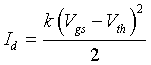(5)

Where K is constant and its value depends uponratio and W is channel width and L is Channel Length.

In a given circuit the two PCS are connected to the Vgs1 and Vgs2 as shown in Figure 6.

The sum of the gate source voltage is kept constant i.e.

Vg = Vgs1 + Vgs2 (6)

And using eq. 1 and eq. 2 two drain current can be given by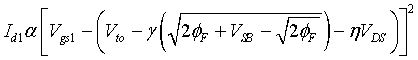(7)

Similarly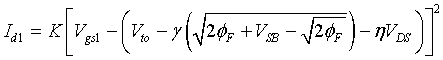(8)

It is observed that the difference Id1-Id2 in the drain currents will depends upon Vgs1 or Vgs2 only, i.e. the device is free from substrate bias effect

Transient analysis

The transient analysis of the device is done on Tanner Tool version 15 and shown in Figure 7 and it is observed that the response is highly linear indicating that the device is stable. On plotting a linear trend line between Vout and Vin the coefficient of determination R2 is found to be 99.7% with standard error of 0.02. The coefficient of determination R2 is useful because it gives the proportion of the variance (fluctuation) of one variable that is predictable from the other variable. It is a measure that allows us to determine how, certain one, can be in making predictions from a certain model. The coefficient of determination is a measure of how well the regression line represents the data. If the regression line passes exactly through every point on the scatter plot, it would be easy to explain all the variations.

Noise analysis

Noise is electrical or electromagnetic energy that reduces the quality of a signal. Noise affects digital, analog and all communications systems. For noise analysis a noise model of the circuit, using noise models of each resistor and semiconductor device is obtained. It calculates the noise contribution of each component and propagates it to the output of the circuit sweeping through the frequency range specified in the analysis dialog box. Noise analysis calculates the noise contribution from each resistor and semiconductor device at the specified output node. Each resistor and semiconductor device is considered a noise generator. Each noise generator’s contribution is calculated and propagated by the appropriate transfer function to the output of the circuit. The total output noise” at the output node is the root mean square (RMS) sum of the individual noise contribution. The result is then divided by the gain from input source to the output source to get the equivalent input noise”. This is the amount of noise which, if injected at the input source into a noiseless circuit, would cause the previously calculated amount of noise at the output. The total output noise” voltage can be referenced to ground or it may be referenced to another node in the circuit. In this case, the total output noise is taken across these two nodes. The onoise and inoise for the given device is shown in Table 1.

Potentiometric Circuit  Integrated Noise Analysis
Onoise_total 0.00876p
Inoise_total 0.00880p

Table 1: Integrated Noise Analysis.

The noise figure is used to specify exactly how noisy a device is. For a transistor, noise figure is simply a measure of how much noise the transistor adds to the signal during the amplification process. In a circuit network, the noise figure is used as a “Figure-of-merit” to compare the noise in a network with the noise in an ideal or noiseless network. It is a measure of the degradation in signal-to-noise ratio (SNR) between the input and output ports of a network. When calculating the noise figure of a circuit design, Noise Factor (F) must also be determined. This is the numerical ratio of noise figure, where noise figure is expressed in dB. Thus,

Noise Figure = 10log10F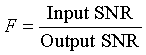The noise figure analysis of the device is observed to be 0.0399db.

Fourier analysis

Fourier analysis is a method of analysing complex periodic waveforms. It permits any non-sinusoidal period function to be resolved into sine or cosine waves and a DC component. This permits further analysis and allows you to determine the effect of combining the waveform with other signals. Each frequency component of the response is produced by the corresponding harmonic of the periodic waveform. Each term is considered a separate source. According to the principle of superposition, the total response is the sum of the responses produced by each term. It is observed that, amplitude of the harmonics decreases progressively as the order of the harmonics increases. This indicates that comparatively few terms yield a good approximation. Fourier analysis of the device is shown in Figure 8.

The comparison between Spice Model and the FIA analysis readings has been shown in Table 2.

→
↓Parameters
Results obtained
from Spice Model
Results obtained
from FIA analysis
Multiple R 0.983 0.958
R2 0.966 0.918
Standard Error 0.026 0.040
Complexities Less complex More complex
Cost Inexpensive Expensive
Accuracy More accurate Less accurate
Behavior Fairly linear Non linear

Table 2: Result comparison.

Inference from Table 2:

a. The value of R2 in case of Spice Model which shows the direction of a linear relationship between peak height decrease in current (ΔI) and dissolved oxygen concentration decrease (ΔO2) is greater compared to FIA model.

b. The value of standard error in Spice Model is found to be smaller which shows better accuracy of the spice model.

c. Spice Model is designed on CAD tools hence it is less complex, inexpensive, fairly linear and more accurate as compared to FIA Model.

#### Result and Conclusion

Various analyses on the given device reveal that the device has fairly good performance. Power analysis on Tanner Tool shows that the device consumes very low power in order of 7.1 mW. The slew rate of the device is good. The output observed in Figure 7 is highly linear, indicating that the device is stable. Coefficient of determination R2 is found to be 99.7% with standard error of 0.02. A significant advantage of the proposed design is that with the use of only few active components and using grounded reference electrode can overcome the problem of using multiple reference electrodes as inputs in an array of sensors. The device has a simple architecture, and hence it is very suitable for water quality monitoring applications. This study may be extended for further improvements in terms of power and size, besides the wiring and layout characteristics level.

#### References

Select your language of interest to view the total content in your interested language

### Article Usage

• Total views: 12149
• [From(publication date):
December-2014 - Jan 23, 2020]
• Breakdown by view type
• HTML page views : 8349Can't read the image? click here to refresh